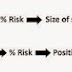## Top Ad unit 728 × 90

A position sizing rule determines the number of contracts or shares that are committed to each trade.
It is very easy to see the impact of position sizing at either extreme.
If we trade a position size that is too small—for example, one S&P con- tract per \$1,000,000—our trading equity is not being used at optimal levels. Conversely, a position size that is too large—for example, 100 S&P per \$100,000—for our capital can increase our risk of ruin to certainty.
Only the most naive and inexperienced of traders will be ensnared by either of these extremes. It is in the area between these two opposite ends of the spectrum, however, where a sizing rule will have the most impact on strategy performance, whether positive or negative.
The best and most efficient sizing principle will be the one that applies our trading capital in an optimal manner so as to achieve maximum returns with an affordable risk.
We will look at four position sizing examples:
2. Martingale
3. Anti-Martingale
4. The Kelly method
A volatility adjusted sizing rule uses the size of the account and the size of the risk assumed per contract per trade.
Definition: Volatility adjusted position sizing determines the number of contracts or shares per trade as a fixed percentage of trading equity divided by the trade risk.
For example, assume:
1. A risk size of 3 percent of equity
2. A risk per contract of \$1,000
3. An account size of \$250,000
The trade unit would be seven contracts and is calculated as follows:
Total Equity to Risk = \$7,500(\$250,000 × .03)
Number of Contracts = 7(\$7,500/\$1,000 = 7.5 rounded down = 7)
An example of a well-known money management rule is the Martingale strategy. It is derived from a gambling money-management method.
Definition:The Martingale sizing rule doubles the trade size after each loss and starts at one unit after each win.
There are a number of variations on this theme. One such variation is anti-Martingale.
Definition: The anti-Martingale sizing rule doubles the number of trading units after each win, and starts at one unit after each loss.
Optimal f (fixed fractional trading) was introduced by Ralph Vince in 1990. It is based on a formula derived from the Kelly method, which was, in turn, applied by Professor Edward Thorpe to gambling and trading.
Let us look at one implementation of the Kelly formula.
Kelly% = (Win% − Loss%)/(Average Profit/Average Loss).Position size
Position SizeReviewed by learn forex trading on November 14, 2014 Rating: 5